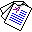# Partial Deduction in Disjunctive Logic Programming

## Chiaki Sakama and Hirohisa Seki

Journal of Logic Programming 32:229-245, 1997.

## Abstract

This paper presents a partial deduction method in disjunctive logic programming. Partial deduction in normal logic programs is based on unfolding between normal clauses, hence it is not applicable to disjunctive logic programs in general. Then we introduce a new partial deduction technique, called disjunctive partial deduction, which preserves the minimal model semantics of positive disjunctive programs and the stable model semantics of normal disjunctive programs. From the procedural side, disjunctive partial deduction is combined with a bottom-up proof procedure of disjunctive logic programs, and top-down partial deduction is introduced for query optimization. Disjunctive partial deduction is also applied to optimizing abductive logic programs and compiling propositional disjunctive programs.Full Paper (pdf 918K)